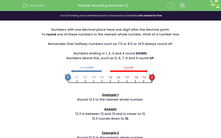# Rounding Decimals (1)

In this worksheet, students must round numbers with one decimal place to the nearest whole number.Key stage:  KS 2

Curriculum topic:   Number: Fractions and Decimals

Curriculum subtopic:   Round One Decimal Place Up/Down

Difficulty level:#### Worksheet Overview

Numbers with one decimal place have one digit after the decimal point.

To round one of these numbers to the nearest whole number, think of a number line.

Remember that halfway numbers such as 7.5 or 8.5 or 14.5 always round UP.

Numbers ending in 1, 2, 3 and 4 round DOWN.

Numbers above this, such as 5, 6, 7, 8 and 9 round UP.Example 1

Round 12.3 to the nearest whole number.

12.3 is between 12 and 13 and is closer to 12.

12.3 rounds down to 12.

Example 2

Round 10.8 to the nearest whole number.

10.8 is between 10 and 11 and is closer to 11.

10.8 rounds up to 11.

Example 3

Round 16.5 to the nearest whole number.